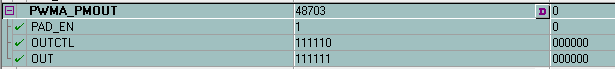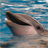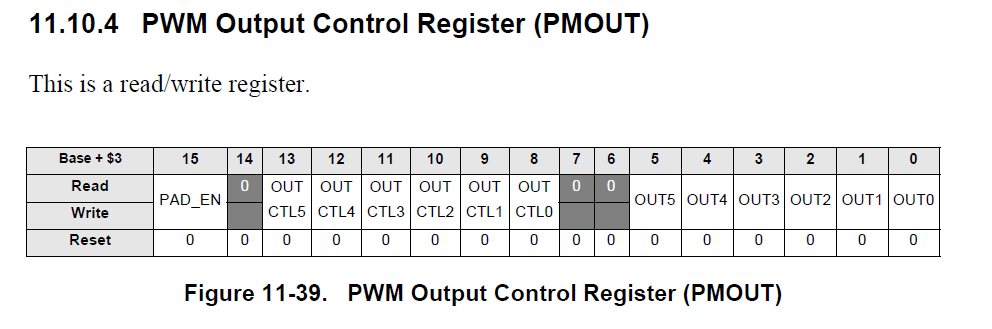## 56F8355 Processor Expert PWMA_PMOUT

cancel
Showing results for
Search instead for
Did you mean:

## 56F8355 Processor Expert PWMA_PMOUT

137 ViewsContributor I

In the CoderWarrrior for 56F8355 DSP under processor expert -> Components -> Timers -> ExcPWM.PWM bean I have the following setting for PWMA_PMOUT.When code is compiled this bean generates code under ExcPWM.c. The function ExcPWM_Init value for PWMA_PMOUT does not match the value assigned in bean for initial values. 48730 vs 16191. Any idea why these two are different. I want to set the "Out Control" for channel "0" (bit 0)to disable.

//Generated code

void ExcPWM_Init(void)
{
RatioStore = (word)655U; /* Store initial value of the ratio */
/* PWMA_PMICCR: ??=0,??=0,??=0,??=0,??=0,??=0,??=0,??=0,??=0,??=0,??=0,??=0,??=0,ICC2=0,ICC1=0,ICC0=0 */
setReg(PWMA_PMICCR,0); /* Set up Internal Correction Control Register */
/* PWMA_PMDISMAP1: DISMAP=0 */
setReg(PWMA_PMDISMAP1,0); /* Set up PWM Disable Mapping Register 1 */
/* PWMA_PMDISMAP2: DISMAP=0 */
setReg(PWMA_PMDISMAP2,0); /* Set up PWM Disable Mapping Register 2 */
/* PWMA_PMOUT: PAD_EN=0,??=0,OUTCTL=63,??=0,??=0,OUT=63 */
setReg(PWMA_PMOUT,16191); /* Set up Output Control Register */
setReg(PWMA_PMDEADTM,0); /* Set up Dead-Time Register */
/* PWMA_PMCCR: ENHA=0,nBX=0,MSK=63,??=0,??=0,VLMODE=0,??=0,SWP45=0,SWP23=0,SWP01=0 */
setReg(PWMA_PMCCR,16128); /* Set up PWM Chanel Control Register */
/* PWMA_PMCFG: ??=0,DBG_EN=0,WAIT_EN=0,EDG=1,??=0,TOPNEG45=0,TOPNEG23=0,TOPNEG01=0,??=0,BOTNEG45=0,BOTNEG23=0,BOTNEG01=0,INDEP45=1,INDEP23=1,INDEP01=1,WP=0 */
setReg(PWMA_PMCFG,4110); /* Set up PWM configure register */
setReg(PWMA_PMCNT,0); /* Reset counter */
EnEvent = TRUE; /* Enable events */
EnUser = TRUE; /* Enable device */
setReg(PWMA_PWMVAL0,300); /* Store initial value to the duty-compare register */
setReg(PWMA_PWMCM,30000); /* and to the period register */
SetPV((byte)1); /* Set prescaler register according to the selected high speed CPU mode */
}

Tags (3)
1 Reply
83 ViewsNXP TechSupport

Hi, Satya,

It seems that this is a PE bug, You set the PAD_EN as 1, set the OUTCTL bits as 6b'111110, set the OUT bits as 6b'111111,

the PMOUT register will be 16b' 1011 1110 0011 1111, it is 0xBE3F, in decimal 48703. In the case, you have to write the PMOUT reg yourself.

Hope it can help you

BR

Xiangjun Rong